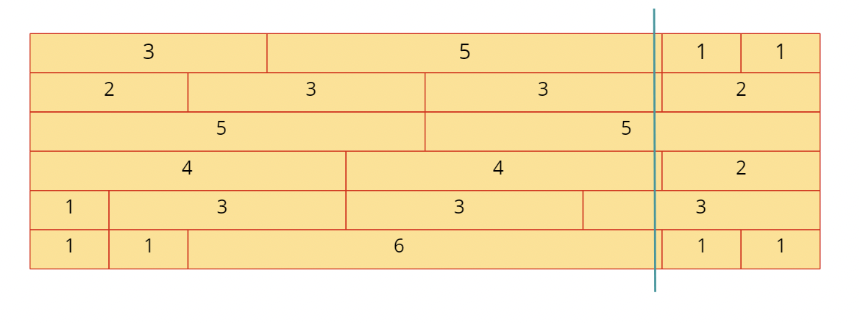# Brick Wall

## 1: U-nderstand

Understand what the interviewer is asking for by using test cases and questions about the problem.

• Established a set (2-3) of test cases to verify their own solution later.
• Established a set (1-2) of edge cases to verify their solution handles complexities.
• Have fully understood the problem and have no clarifying questions.
• Have you verified any Time/Space Constraints for this problem?
• What if there is a tie for the least number of bricks to cross?
• Both values should be the same, so returning either would be the same value as well.
• Could the input array be empty?
• It could. In that case let’s try to return 0 because we wouldn’t cross any bricks.
• What are the Time/Space Constraints Considerations?
• Try to seek different uses of O(N) space to solve this problem!Source: Medium

Run through a set of example cases:

``````HAPPY CASE
RAW         DIAGRAM
Input: [[1, 2, 3],  |x|xx|xxx|
[1, 3, 2],  |x|xxx|xx|
[4, 1, 1]   |xxxx|x|x|
]
Output: 1 (The best case is that a line would cross 2 gaps between bricks,
so 1 brick.)

HAPPY CASE
RAW         DIAGRAM
Input: [,        |x x x|
[1, 1, 1],  |x|x|x|
[2, 1]      |xx |x|
]
Output: 1

EDGE CASE
RAW         DIAGRAM
Input: [,        |xxx|
,        |xxx|
         |xxx|
]
Output: 3 (A line cannot be drawn at either of the ends, so it must go through
all 3 bricks.)``````

## 2: M-atch

Match what this problem looks like to known categories of problems, e.g. Linked List or Dynamic Programming, and strategies or patterns in those categories.

• Stack: What could we push onto a stack to make this problem easier? Stacks don’t allow us to keep track of data based on keys.
• Queue: Queues fall into the same category as Stacks, do we need to maintain any sense of ordering to solve this problem?
• HashMap: HashMaps allow us to store data for quick access. What could we store in a HashMap to make this problem easier?
• Heap: Do we need some sort of ordering to our data that a Heap could provide?

## 3: P-lan

Plan the solution with appropriate visualizations and pseudocode.

General Idea: Use a HashMap to store number of gaps at column indices. Then, use the max gap value to calculate the least number of bricks to cross in a vertical line.

``````1. Create a HashMap to store the number of gaps at each index of a row.
2. Iterate through each row within the wall
3. Create a sum variable
4. Iterate through the row, besides the last index
a) At each point calculate the sum up until that index
b) Index into the current sum key within the HashMap
c) Increment that value to indicate there is one more gap at that index
5. Calculate the index with the largest number of gaps from the HashMap
a) The largest 'value' within the HashMap indicates the index with the
highest number of gaps
6. Return the height of the wall minus the highest gap value
- This means the number of bricks you will cross in creating a line there``````

⚠️ Common Mistakes

• Some people may try to approach this problem initially with some brute force O(N^2) strategy. However, this approach doesn’t work. Try to urge students to avoid usual brute force tactics. Instead, urge them to use known data structures to solve this problem.

## 4: I-mplement

Implement the code to solve the algorithm.

``````int leastBricks(vector<vector<int>>& wall) {
int rows = size(wall), maxBrickEdges = 0, idx;
unordered_map<int, int> edgesFrequency; // will map index to count of brick edges at that index
for(auto& row : wall) {
// idx: denotes index of wall. add each brick-width of row to find the next index having brick edge
idx = 0;
for(int i = 0; i < size(row) - 1; i++) // ignore last brick, since we don't want to count wall edge index
idx += row[i], edgesFrequency[idx]++;
}
// lastly find the maximum number of brickEdges found at any index
for(auto& pair : edgesFrequency) maxBrickEdges = max(maxBrickEdges, pair.second);
// rest of the bricks(excluding maxBrickEdges) would be intersected which is the minimum answer
return rows - maxBrickEdges;
}``````
``````public int leastBricks(List<List<Integer>> wall) {
Map<Integer, Integer> freq = new HashMap<>();
// the line can be drawn where max rows have brick ends
// so calculate the freq of each brick end across rows
for (List<Integer> row : wall) {
int end = 0;
for (int i = 0; i < row.size() - 1; i++) {
end += row.get(i);
freq.put(end, freq.getOrDefault(end, 0) + 1);
}
}

// calculate the brick-end with max freq
int max = 0;
for (Map.Entry<Integer, Integer> entry : freq.entrySet()) {
int val = entry.getValue();
if (val > max) max = val;
}

// the #bricks-crossed is the #rows where the brick-end w/ max freq is not present
// i.e. it has got a brick there.
return (wall.size() - max);
}``````

## 5: R-eview

Review the code by running specific example(s) and recording values (watchlist) of your code's variables along the way.

• Trace through your code with an input to check for the expected output
• Catch possible edge cases and off-by-one errors

## 6: E-valuate

Evaluate the performance of your algorithm and state any strong/weak or future potential work.

• Time Complexity: `O(R*W)`, where `R` is the number of rows in brick wall and `W` is the width of each row.
• Space Complexity: `O(W)`, required to maintain hashmap.